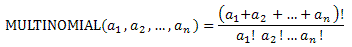## Excel Office

Excel How Tos, Tutorials, Tips & Tricks, Shortcuts

# MULTINOMIAL function: Description, Usage, Syntax, Examples and Explanation

## What is MULTINOMIAL function in Excel?

MULTINOMIAL function is one of the Math and Trig functions in Microsoft Excel that returns the ratio of the factorial of a sum of values to the product of factorials.

## Syntax of MULTINOMIAL function

MULTINOMIAL(number1, [number2], …)

The MULTINOMIAL function syntax has the following arguments:

• Number1, number2, …    Number1 is required, subsequent numbers are optional. 1 to 255 values for which you want the multinomial.

## MULTINOMIAL formula explanation

• If any argument is nonnumeric, MULTINOMIAL returns the #VALUE! error value.
• If any argument is less than zero, MULTINOMIAL returns the #NUM! error value.
• The multinomial is:## Example of MULTINOMIAL function

Steps to follow:

1. Open a new Excel worksheet.

2. Copy data in the following table below and paste it in cell A1

Note: For formulas to show results, select them, press F2 key on your keyboard and then press Enter.

You can adjust the column widths to see all the data, if need be.

 Formula Description Result =MULTINOMIAL(2, 3, 4) Ratio of the factorial of the sum of 2,3, and 4 (362880) to the product of the factorials of 2,3, and 4 (288). 1260